Effect of Temperature Inversion on Lower Nodes

October 28, 2020

Story

The threshold voltage is the minimum voltage required for the flow of electrons through the channel. It is denoted by VTH of a MOSFET.

The threshold voltage is the minimum voltage required for the flow of electrons through the channel. It is denoted by VTH of a MOSFET. Further, it’s the value of the gate-source voltage when the conducting channel is just ready to connect to the source and drain contacts of the transistor. This allows for significant current to flow. Mobility is the measurement of how fast the carriers (electrons or holes) can move through a semiconductor in the presence of an electrical field. Semiconductor mobility depends on the impurity concentrations, temperature, electrons, and holes concentrations. Mobility is the proportionality constant between an electric field and the drift velocity of the carriers in the conductive material. Depending on the quality of a semiconductor crystal, the number of impurities in the crystal, and the temperature.

The Effect of temp on mobility, threshold voltage, and their effect on the delay of HVT and LVT cells.

As temperature increases, lattice vibrations increase, and the probability of an electron being scattered by the lattice increases. Thus, in high temperatures, mobility is limited by phonon scattering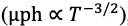, causing mobility to decrease.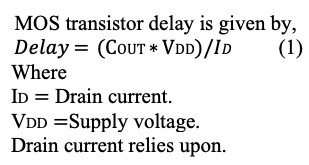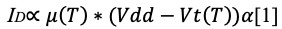Where μ represents mobility and VTH represents threshold voltage. 1 ≤α ≥ 2

ID depends on Mobility (µ) and threshold voltage (VTH). The drain current is decided by the factor that influences it at a given temperature and voltage. Mobility decide the drain current at high supply voltages (VDD).

At lower supply voltage (VDD) threshold voltage ( VT) influence and determines the drain current.

Threshold voltage

The MOSFET threshold voltage is given by;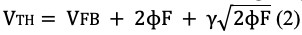Where VFB is the ?at band voltage, γ is the body effect parameter of Si, and is the Fermi energy. The threshold voltage in a MOSFET is commonly modeled to decrease linearly with increasing temperature.

The final drain current is decided by two factors: threshold voltage ( VT) and mobility (µ). Whichever influences the drain current at a given temperature and supply voltage. At a high VDD, the mobility (µ) decides the drain current, but at a low VDD, the threshold voltage decides the drain current.

• At a high supply voltage, higher temperature increases the delay.
• At a low supply voltage, higher temperature decreases the delay.

Thus, the delay increases or decreases with increasing temperature depending on the magnitude of the supply voltage (VDD).

Temperature inversion effects on delay of cell

As mentioned, both the mobility and the threshold voltage decrease with increasing temperature. However, their effect on the drain current is the reversed.

• Lower mobility reduces the drain current.
• Lower threshold voltage results in increment in the (ID) drain current.

So, it can be stated that as the temperature increases the delay of cell changes according to change in current (ID) stated in equation 1.

• Decrement in threshold voltage (VTH) resulting in reduction in cell delay.
• Lowering in mobility resulting in increment in cell delay.

Threshold voltage (VTH) and mobility (µ) whichever will be dominant will determine the cell delay whether delay of cell will rise or fall.

CASE 1: When the (VDD -VTH) gate over-drive voltage is high, then there is negligible variation in threshold voltage due to temperature variation (fig 1). Effects of variation in Threshold voltage is very small in delay. The mobility effect dominates, due to the delay of the cell increases with increases in temperature.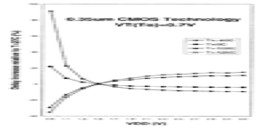(Fig1)

CASE 2: When VDD is low, its value approaches towards VTH. At lower nodes the threshold voltage (VTH) has not been reduced by much, but the supply voltage has reduced considerably to lower the leakage power concerns (fig 2). Then, threshold voltage variation VTH has a dominant affect on the delay.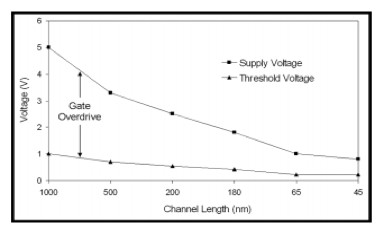(Fig 2)

At Lower VDD and higher temperatures the value of cell delays decrease as VDD approaches to VTH.

Table 1 and Table 2 contains delays of 180nm and 45nm cells, two different temperatures (0°C and 125°C), at two different supply voltages (1.8 and .8v). It observed that delay of cells increases. When (VDD -VTH) gate, over-drive voltage is high. Then, there is negligible variations in threshold voltage due to temperature variation being inadequate. Hence, the effect of variation in threshold voltage is very small which results in small change in cell delay.

The mobility effect dominates, due to the delay of the cell increase in temperature as shown in Table 1.

When VDD is low its value approaches to VTH. So, at lower nodes the threshold voltage (VTH) has not been reduced, but the supply voltage has decreased the threshold voltage variation.

VTH have a dominant effect on the delay due to which the delay of the cell decreases with increases in temperature as shown in Table 2.

DELAY VARIATION WITH TEMPERATURE FOR CIRCUITS OPERATING AT THE NOMINAL SUPPLY VOLTAGE (V = 1.8 V) IN A 180-nm CMOS TECHNOLOGY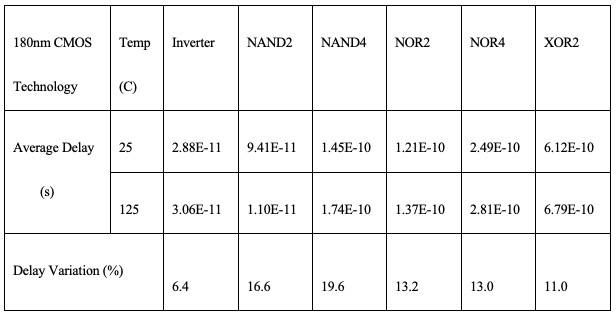(Table 1)

DELAY VARIATION WITH TEMPERATURE FOR CIRCUITS OPERATING AT THE NOMINAL SUPPLY VOLTAGE (V = 0.8 V) IN A 45-nm CMOS TECHNOLOGY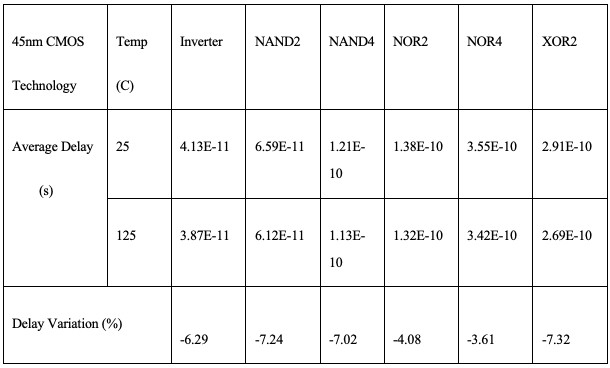(Table 2)

According to the experiment done on 16nm result

The temperature inversion effect is more prominent on higher Vt cells at lower supply voltage.

The effect on high VTH cells responding to temperature inversion is more effective. SVT does not show much variation and LVT Variations are minimum as shown in Table 3 done on 16nm technology node.

DELAY VARIATION WITH TEMPERATURE 125AND OPERATING AT THE NOMINAL SUPPLY VOLTAGE (V = 0.8 V) in 16-nm TECHNOLOGY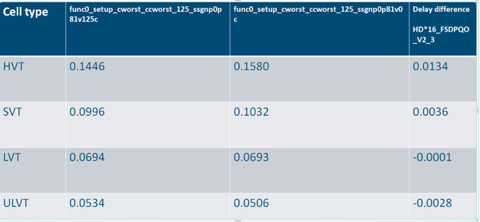(Table 3)

Observation

In lower technology nodes, the supply voltage is less and the effect of temperature inversion can be seen. It can be seen in the above experiment, showing cell delay is decreasing with an increase in temperature. Therefore, the effect of VTH (threshold voltage) is more and mobility contribution is small on cell delay as Temperature increases.

Thus, for low in lower technologies, the cell delay decreases when temperature increases, the cell delay shows an opposite behavior to the temperature at lower node technologies as compared to higher technology nodes.

Conclusion

Temperature inversion effects mobility and threshold voltage, and these factors are dependent on the supply voltage. If the supply voltage is in the range which is comparable to VTH then the threshold voltage effect is dominant, whereas if the supply voltage is larger than VTH then mobility has the dominant effect.

References

    S. W. Sun and P. G. Y. Tsui, “Limitation of CMOS Supply-Voltage Scaling by MOSFET Threshold-Voltage Variation,” IEEE J. Solid-State Circuits, 1995, doi: 10.1109/4.400439.

    S. Sze, “Physics of semiconductor devices 2nd edition,” Microelectronics J., 1981, doi: 10.1016/S0026-2692(82)80036-0.

    A. Bellaouar, A. Fridi, M. I. Elmasry, and K. Itoh, “Supply voltage scaling for temperature insensitive CMOS circuit operation,” IEEE Trans. Circuits Syst. II Analog Digit. Signal Process., 1998, doi: 10.1109/82.664253.

    R. Kumar and V. Kursun, “Reversed Temperature-Dependent Propagation Delay Characteristics in Nanometer CMOS Circuits,” IEEE Trans. Circuits Syst. II Express Briefs, 2006, doi: 10.1109/TCSII.2006.882218.

     http://robertdick.org/talp/papers/dasdan-temperature.pdf

Dr. Rajendra Pratap

M.Tech and B.Tech from IIT.

Sandeep Mall

B.tech Electronics and communication